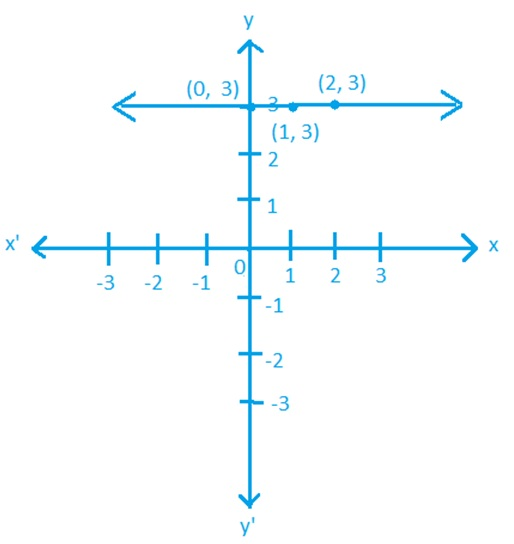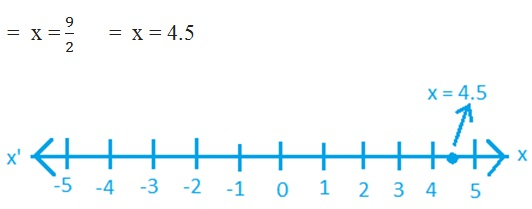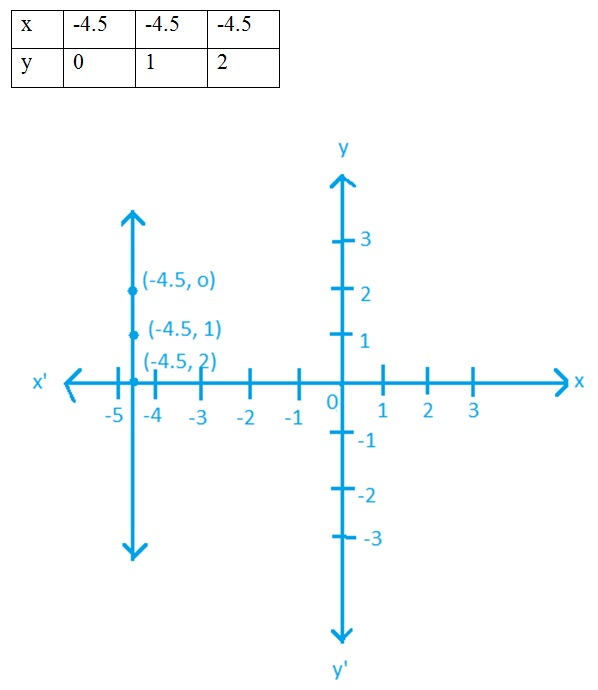Study Materials

# NCERT Solutions for Class 9th Mathematics

Page 4 of 4

## Chapter 4. Linear Equation In Two Variables

### Exercise 4.4

Linear equation in two variable

Exercise : 4.4

1. Give the geometric representations of y = 3 as an equation :

(i) in one variable

(ii) in two variables

Solution :

(i) y = 3(ii) y = 3

=   (0)x + y = 3

=   y = 3 - (0)x2. Give the geometric representations of 2x + 9 = 0 as an equation :

(i) in one variable

(ii) in two variables

Solution :

(i) 2x + 9 = 0(ii) 2x + y = 0

=   2x + 0.y + 9 = 0

=   2x = -9      =  x = -4.5Page 4 of 4

Chapter Contents: